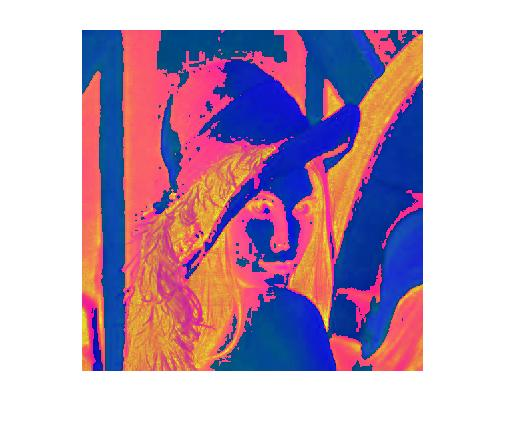MATLAB中RGB图像转HSI图像的源代码，并图像分割 2C

MATLAB中RGB图像转HSI图像的源代码

2个回答

`````` function hsi = rgb2hsi(rgb)
% hsi = rgb2hsi(rgb)把一幅RGB图像转换为HSI图像，
% 输入图像是一个彩色像素的M×N×3的数组，
% 其中每一个彩色像素都在特定空间位置的彩色图像中对应红、绿、蓝三个分量。
% 假如所有的RGB分量是均衡的，那么HSI转换就是未定义的。
% 输入图像可能是double（取值范围是[0, 1]），uint8或 uint16。
%
% 输出HSI图像是double，
% 其中hsi(:, :, 1)是色度分量，它的范围是除以2*pi后的[0, 1]；
% hsi(:, :, 2)是饱和度分量，范围是[0, 1]；
% hsi(:, :, 3)是亮度分量，范围是[0, 1]。

% 抽取图像分量
rgb = im2double(rgb);
r = rgb(:, :, 1);
g = rgb(:, :, 2);
b = rgb(:, :, 3);

% 执行转换方程
num = 0.5*((r - g) + (r - b));
den = sqrt((r - g).^2 + (r - b).*(g - b));
theta = acos(num./(den + eps)); %防止除数为0

H = theta;
H(b > g) = 2*pi - H(b > g);
H = H/(2*pi);

num = min(min(r, g), b);
den = r + g + b;
den(den == 0) = eps; %防止除数为0
S = 1 - 3.* num./den;

H(S == 0) = 0;

I = (r + g + b)/3;

% 将3个分量联合成为一个HSI图像
hsi = cat(3, H, S, I);
%hsi = H;    %分别返回3个分量
%hsi = S;
%hsi = I;
``````